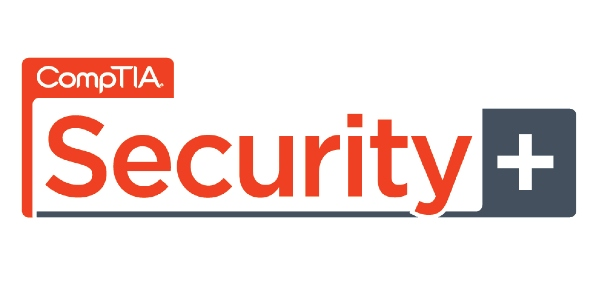# Set3 CompTIA Security+ Sample Questions: Cryptography

23 Questions | Total Attempts: 6307SettingsThis is Set 3 of 4 of practice questions for CompTIA Security+ exam. This set covers the exam objective Cryptography. You can take these Security+ sample questions for practice or take it as a topic wise practice exam. Read the questions carefully and answer. So, let's try out the quiz. All the best! Feedback and comments are welcome.

Related Topics
• 1.
• A.

SHA-1

• B.

SHA-4.1

• C.

SHA-4

• D.

None of the above

• 2.
Choose the odd one out.
• A.

RC5

• B.

Blowfish

• C.

MAC

• D.

ECC

• 3.
• A.

Public key

• B.

Private key

• C.

Session key

• D.

Session ID

• 4.
ECC is the encryption system used in cellular devices. T/F?
• A.

True

• B.

False

• 5.
Cryptography is an essential in encapsulation protocols. T/F?
• A.

True

• B.

False

• 6.
• A.

The number of keys used by the encryption protocol

• B.

The number of keys used by the algorithm

• C.

Number of bits used for encryption by the algorithm

• D.

None of the above

• 7.
Cryptanalysis is nothing but pattern finding T/F?
• A.

True

• B.

False

• 8.
• A.

True

• B.

False

• 9.
• A.

It uses an offset value of 3 for sliding alphabets

• B.

There is a difference of 3 between the alphabets when the sliding action occurs

• C.

It uses 3 keys for encryption

• D.

None of the above

• 10.
Which of the following is an advantage of using conventional encryption?
• A.

It is the most secure

• B.

It is very fast

• C.

It is economical

• D.

None of the above

• 11.
The concept of public key cryptography was introduced by Julius Caesar. T/F?
• A.

True

• B.

False

• 12.
• A.

PK cryptography is a very complex and nearly impossible setup

• B.

You need just one single key for encrypting as well as decrypting in PK cryptography

• C.

Public key can only encrypt and private key can only decrypt

• D.

None of the above

• 13.
The higher the number of bits, more secure is the key. T/F?
• A.

True

• B.

False

• 14.
A digital signature is used for which of the following?
• A.

Encrypting

• B.

Identifying

• C.

Encapsulating

• D.

None of the above

• 15.
• A.

Hash

• B.

Digital signature

• C.

Token

• D.

None of the above

• 16.
Even today only upto 20% of critical information being transmitted is really secure. T/F?
• A.

True

• B.

False

• 17.
PKI uses which of the following to ascertain identity?
• A.

Certificate

• B.

Digital signature

• C.

Hand print

• D.

None of the above

• 18.
PKI requires skilled and continuous maintenance on the network. T/F?
• A.

True

• B.

False

• 19.
Traditional PKI uses self assembly process to maintain security. T/F?
• A.

True

• B.

False

• 20.
Which of the following is an improvement over PKI?
• A.

Certificates

• B.

SMSA

• C.

Token

• D.

None of the above

• 21.
Which of the following statements is true?
• A.

A private key may be derived from a public key

• B.

Given a public key it is very difficult to derive the private key

• C.

A session ID is used to create a public key

• D.

None of the above

• 22.
Which of the following is an improvement over the scheme used in digital signatures?
• A.

Token

• B.

One-way hash functions

• C.

Cryptanalysis

• D.

None of the above

• 23.
Which of the following is used to sign the certificate created by the CA?
• A.

CA’s digital signature

• B.

CA’s private key

• C.

CA’s certificate

• D.

None of the above